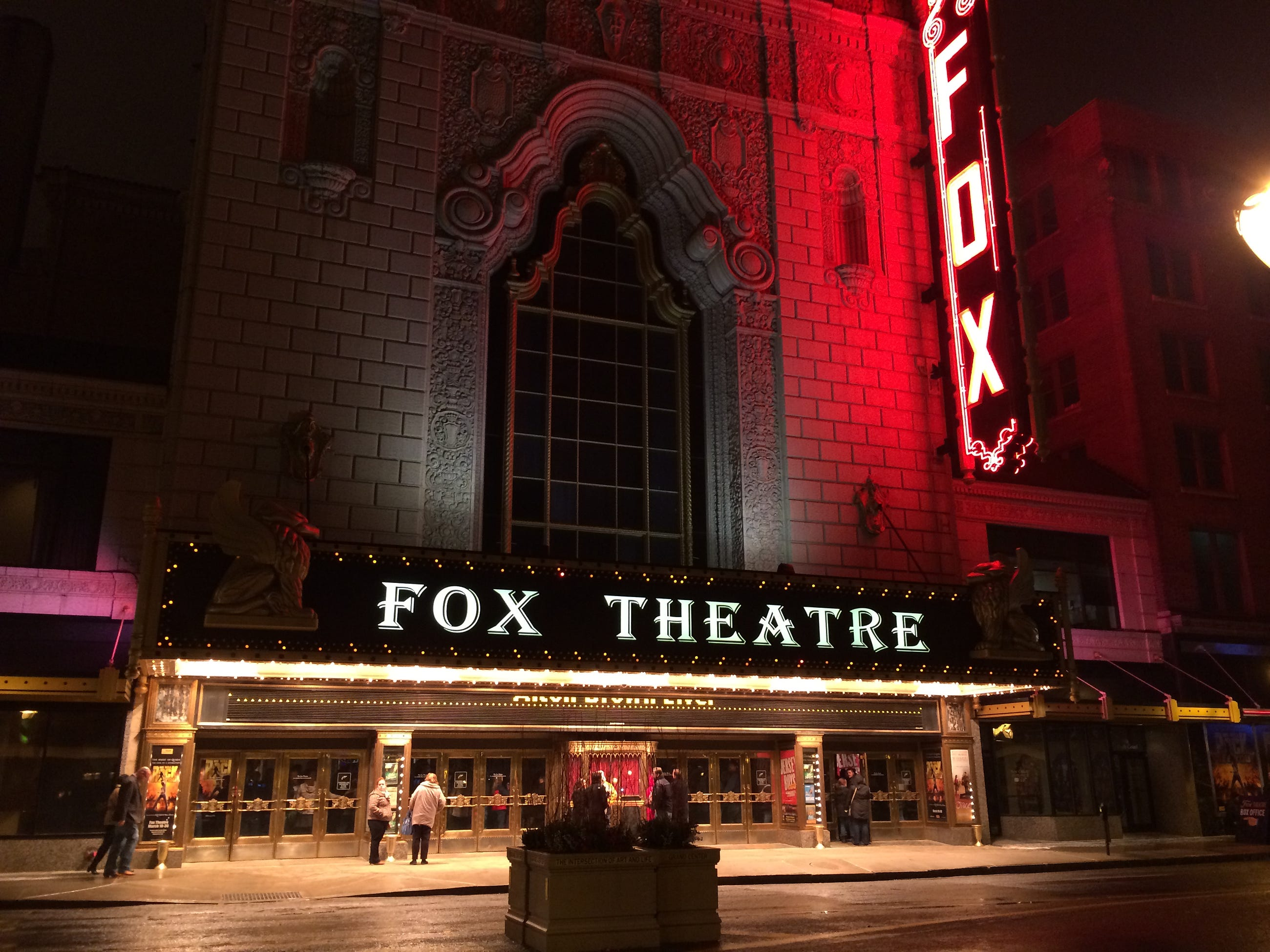# Celebrity Face Generation With Deep Convolutional GANs

Original article was published by NVS Yashwanth on Deep Learning on Medium## Large-scale CelebFaces Attributes (celebA) dataset

CelebFaces Attributes Dataset (CelebA) is a large-scale face attributes dataset with more than 200K celebrity images, each with 40 attribute annotations.

We don’t need the annotations so we will have to crop images. Now, these are color images. Thus, depth is 3 (RGB — 3 color channels).

We can resize & crop the images to a size of 32×32. This can be later converted into tensors.

Note that we user image folder wrapper function here.

## Visualizing our training data

We will now generate a batch of data and visualize the same. Please note that the np.transpose function translates the image dimension by the order specified. For example, an RGB image of shape 3x32x32 gets transposed to 32x32x3 upon calling the following function:

`np.transpose(img,(1,2,0))`

Our batch size is 128, so instead of plotting all 128 images of a batch, we only plot 20 in this case.

## Scaling images

It is important to scale the images as the Tanh function has values in the range -1 to 1, so we need to rescale our training images to a range of -1 to 1. (Right now, they are in a range from 0-1.)

## Helper functions — Convolutional & Transpose Convolutional

To make things easier and simply our code, we will define helper functions for defining our Discriminator & Generator networks.

The reason for these helper functions? Answer — DRY! 😅

Convolution helper function

Transpose Convolution helper function

Note: If you want to read about transpose convolution, check out the article below.

## Discriminator Architecture

We shall now define our discriminator network. The discriminator as we know is responsible for classifying the images as real or fake. Thus this is a typical classifier network.

## Generator Architecture

The generator network is responsible for generating fake images that could fool the discriminator network into being classified as real. Over time the generator becomes pretty good in fooling the discriminator.

## Parameter initialization

We will initialize the weights and biases of our network by sampling random values from a normal distribution. This results in better results. We define a function for the same that takes a layer as an input.

For weights, I used 0 mean and 0.02 standard deviation.

For bias, I used 0.

Now this should be in place replacement so the _(underscore) after the function does the same.

## Loss functions and optimizer

We shall make use of Adam optimizer with a learning rate of 0.002. This is as per the original research paper on DCGANs. You can check it out below.

We used BCEwithLogitsLoss(), which combines a sigmoid activation function (we want the discriminator to output a value 0–1 indicating whether an image is real or fake) and binary cross-entropy loss.

## Training phase

We will define a function for training our model. The parameters would be the Discriminator, Generator, epoch number.

## Sample generation

Let us generate a few samples now. It is important that we rescale the values back to the pixel range(0–255).

And finally, we have our generated faces below. 👀

Well, that is pretty good for a small network aye!

## Takeaways

1. A human face contains multiple features and some of the features are very complicated such as freckles and facial hair. To generate proper images we might need images with high resolution.
2. Provided with the high-resolution images for training we might need to build a better and deeper model for better accuracies.
3. Based on the input image we can further increase the depth and number of layers of the model.
4. Increasing the resolution can surely help us improve the model and capture more features precisely.
5. The samples generated can be further improved by tweaking the parameters such as learning rate, batch size, and training for more number of epochs. The loss of the generator is fluctuating and is not decreasing.
6. The CelebA data mostly contains images of different celebrities at different angles and lighting conditions.